# Riemann Hypothesis: What Yitang Zhang’s New Paper Means and Why You Should Care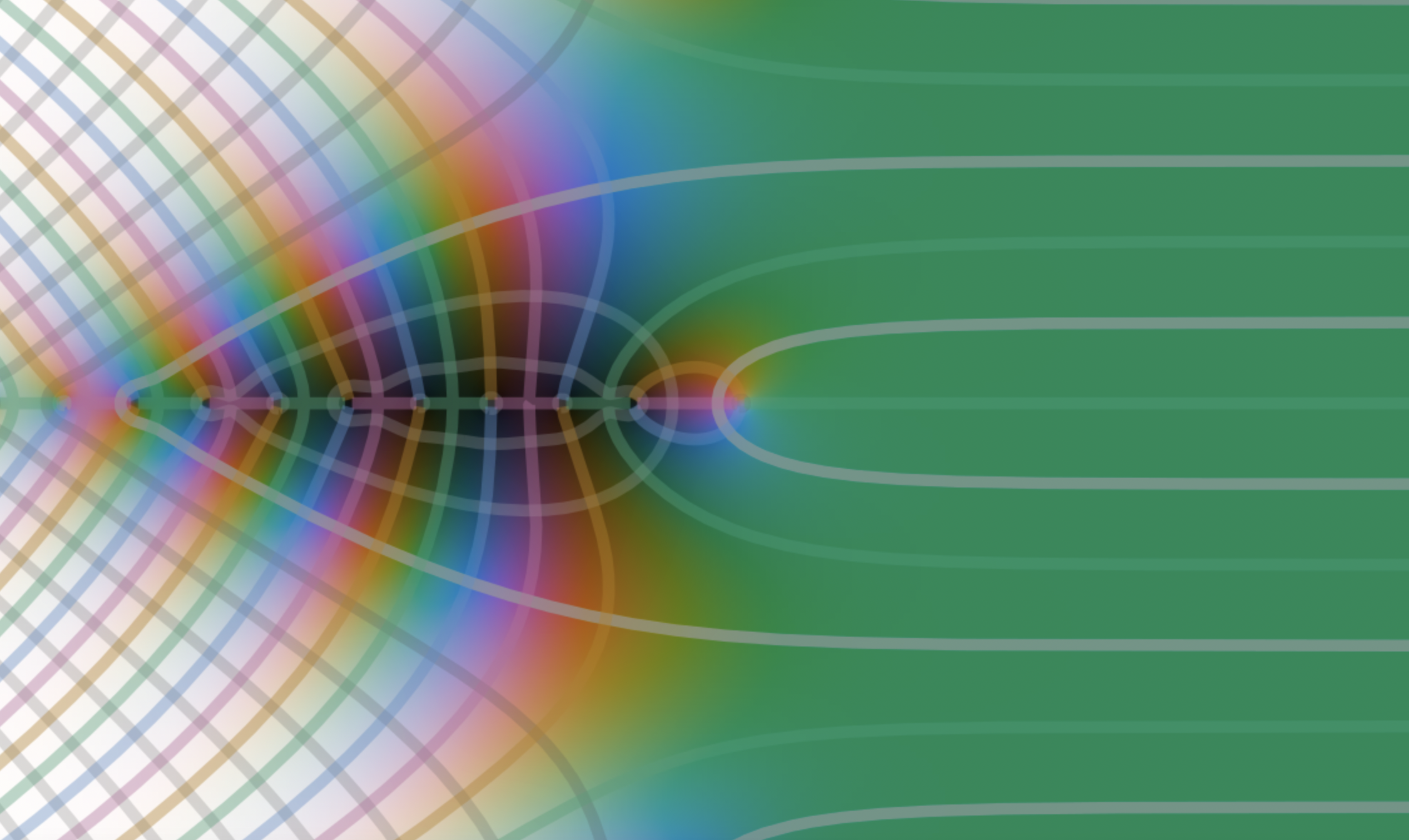A part of a visualisation of the Riemann zeta function in the complex plane. The Re(½) line passes vertically through the middle. Plot: Nschlow/Wikimedia Commons, CC BY-SA 4.0

• Euclid found long ago that there are infinitely many prime numbers. However, they are not distributed evenly: they become less common as they become larger.
• The Riemann hypothesis makes an important statement about their distribution, offering to remove the seeming arbitrariness with which they turn up and impose order.
• The hypothesis is about the form that solutions to the Riemann zeta function, which could estimate the number of prime numbers between two numbers, are allowed to take.
• Yitang Zhang has claimed that he has disproved a weaker version of the Landau-Siegel zeroes conjecture, an important problem related to the hypothesis.
• The conjecture is that there are solutions to the zeta function that don’t assume the form prescribed by the Riemann hypothesis.

Earlier this October, Chinese websites claimed that the Chinese-American mathematician Yitang Zhang had solved the Landau-Seigel zeros conjecture – an important open problem in number theory related to cracking a bigger problem: is there a pattern to the way prime numbers are distributed on the number line?

It’s a simple question but it has only complicated answers. While mathematicians pursuing a resolution to the hypothesis may be motivated by their quest for knowledge alone, many others – including physicists – are interested because the answer has tantalising connections to many concepts in modern physics.

In 1859, the German mathematician Bernhard Riemann came close to answering the question when he formulated the Riemann hypothesis. It expressed an idea about a function he had discovered, called the Riemann zeta function, and its ability to estimate the number of prime numbers up to a particular point on the number line.

The Riemann hypothesis is often considered the most important unsolved problem in pure mathematics today. And Yitang Zhang has claimed that he has taken a big step towards solving it. Has he?

The zeta function

Prime numbers are the basic building blocks of natural numbers. Think of prime numbers as what atoms are to matter, or what alphabets are to a language. If you can create a periodic table of prime numbers, you will have a way to understand all numbers.

The Greek mathematician Euclid found long ago that there are infinitely many prime numbers. In the millennia since, we have learnt that 2 is the first prime1, 3 is the second prime, 5 is the third prime, 7 is the fourth prime, 11 is the fifth prime and so forth.

However, the prime numbers are not distributed evenly. They become less common as they become larger. For example:

* 40% of numbers up to 10 are prime numbers,

* 25% of numbers up to 100 are prime numbers,

* 16.8% of numbers up to 1,000 are prime numbers,

* 12.3% of numbers up to 10,000 are prime numbers,

* 9.6% of numbers up to 100,000 are prime numbers,

* 7.8% of numbers up to 1,000,000 are prime numbers,

… and so on.

But mathematicians don’t think this distribution is entirely arbitrary; they believe there could be a pattern. An important stop on the quest for this pattern is the prime number theorem.

Let’s start with the prime-counting function. Its graph (shown below) is that of a counter: flat when there is no prime number, +1 when there is a prime number. It is denoted as π(x). x here is the position on the real number line. The fraction of prime numbers up to x is π(x)/x.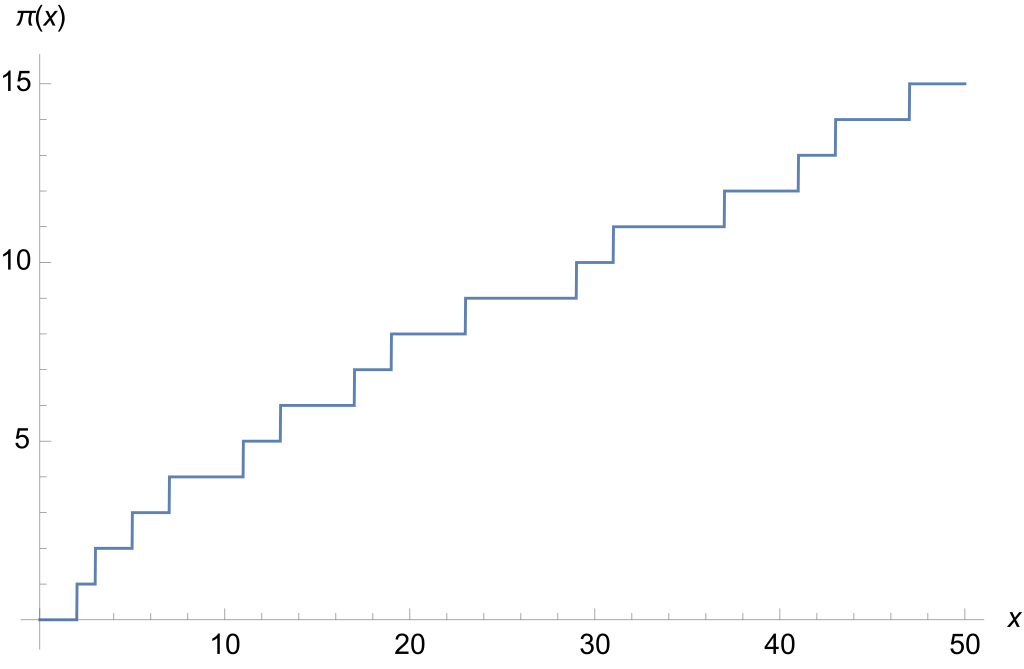A plot of the prime counting function up to 50. Plot: Googolplexian1221/Wikimedia Commons, CC BY-SA 4.0

In the late 1700s, when he was still a teenager, the German mathematician Carl Friedrich Gauss had an idea: that for large values of x, the value of the prime counting function π(x) will be approximately equal to that of the logarithmic integral function. In other words, at a point far along the number line, the total number of prime numbers until that point will get closer and closer to the value of the function shown below: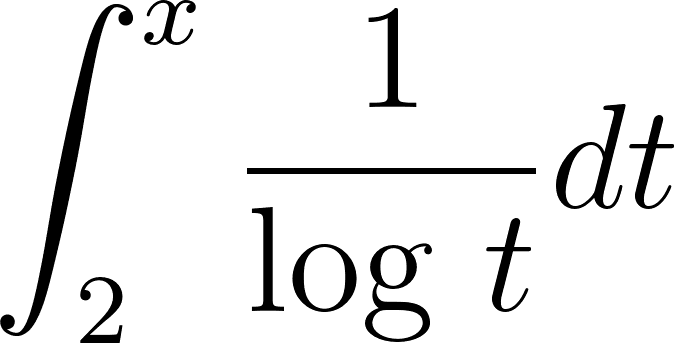In yet other words, at position x, the ratio of the number of prime numbers to x will be roughly equal to 1/log x.

This is the prime number theorem as formulated by Gauss.

In the 1850s, Riemann – who was a student of Gauss – investigated Gauss’s conjecture. He discovered a profound connection between the conjecture and the zeta function – another function that had been investigated by the famous Swiss mathematician Leonard Euler a century earlier.

The zeta function is an infinite sum of the following form: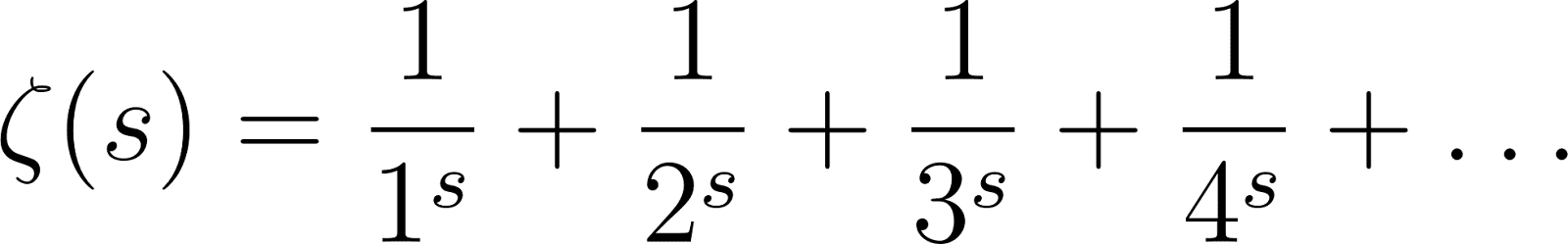Euler had proved that when s > 1, the sum ζ(s) has a finite value. He found that ζ(2) is equal to π2/6…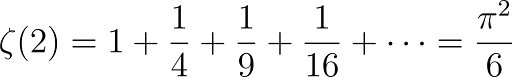… and that ζ(4) is equal to π4/90.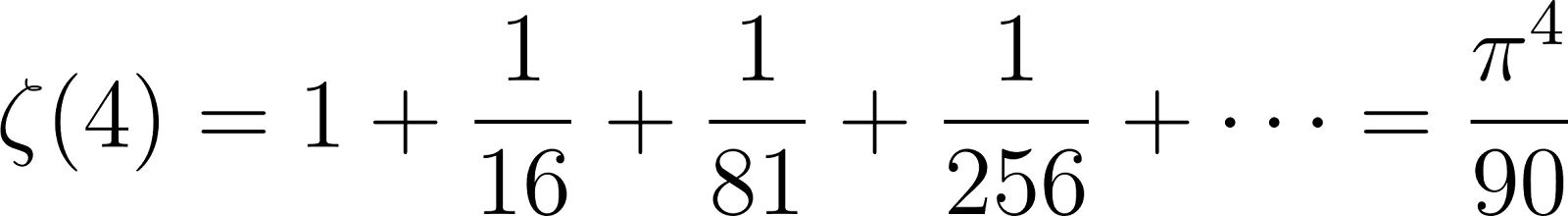Euler also found that the zeta function, which is expressed as an infinite sum, could be expressed as an infinite product: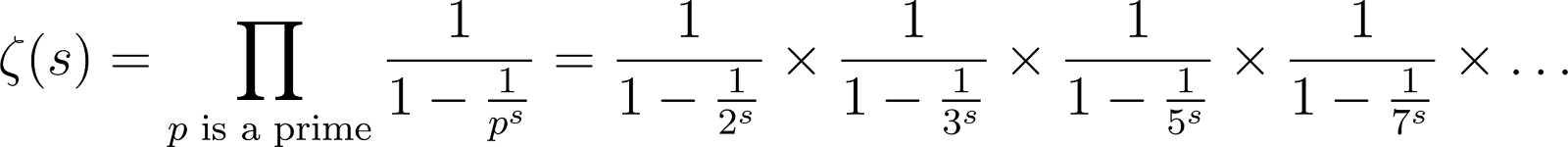The Riemann hypothesis

Riemann studied the zeta function using a branch of mathematics he pioneered called complex analysis. Specifically, he used a technique called analytic continuation to make sense of the values of the zeta function for complex inputs.

That is, he found a way to calculate the value of ζ(s) when s is a complex number.

A complex number is any number of the form a + bi. Here, a and b are real numbers and i is the imaginary unit: i = √-1.

Riemann observed that in the new domain of complex numbers, for some values of s, the value of ζ(s) was 0. These values of s are called the zeta zeroes. Some of them were easier to find. For example, for every negative even integer s (-2, -4, -6, …), ζ(s) equals 0. Riemann called these the trivial zeros. (-2 is not a complex number but can be expressed as one: -2 + 0i.)

There are other zeta zeroes called the non-trivial zeroes – they form the crux of the Riemann hypothesis. Riemann could show that on a graph, all the non-trivial zeroes should lie in a region called the critical strip – between the vertical lines passing through 0 and 1 on the x-axis (see below).

The Riemann hypothesis is the statement that all the non-trivial zeroes should lie not just somewhere in the strip but on a single vertical line called the critical line, which passes through 1/2 on the x-axis.

In technical terms

Recall that Riemann’s first insight was a connection between Gauss’s conjecture and the zeta function. Gauss’s conjecture stated that the value of π(x) for a large x is roughly equal to the value of a different function.

Riemann found this connection when he modified the prime-counting function π(x) and arrived at a new formula, J(x):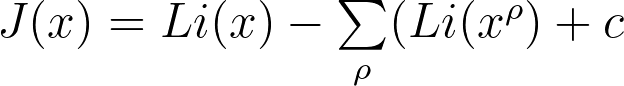The first term, Li(x), approximates Gauss’s original prime counting function, π(x). ‘Li’ stands for ‘logarithmic integral’. The second term is the sum of the logarithmic integral of x to the power ρ2, and summed over ρ. The ρ denotes the non-trivial zeroes of the zeta function. The term c is a constant.

Say the non-trivial zeroes are the complex numbers a, b, c, d and e. The sum will then be over a to e: (Li(xa)) + (Li(xb)) + (Li(xc)) + (Li(xd)) + (Li(xe)), and finally with the addition of the constant.

The more non-trivial zeroes the function sums over, the tighter its estimate of the prime count will be. That is, the estimate after summing over a thousand zeroes will be better than the estimate after summing over a hundred zeroes.

Also read: Beyond the Surface of Einstein’s Relativity Lay Riemann’s Chimerical Geometry

The form of this function revealed to Riemann that the locations of prime numbers are deeply connected to the locations of the non-trivial zeroes of the zeta function. More specifically, Riemann used the formula to hypothesise that the complex-number representation of each non-trivial zero always had the form:

½ + <a real number> times i

In technical parlance, the Riemann hypothesis states that “the nontrivial zeros of ζ(s) lie on the line Re(s) = ½”.

Jacques Hadamard and Charles Poussin proved Gauss’s conjecture independently in the 1890s using Riemann’s work on the zeta function. Their result is now called the prime number theorem (which we encountered earlier). However, Riemann’s hypothesis itself continues to resist attempts to prove to this day.

Landau-Siegel zeroes

An arithmetic progression is a sequence of numbers where the next term is the previous term plus a constant. For example, 1, 3, 5, 7, 9… is an arithmetic progression where the constant is +2.

In 1837, the German mathematician P.G.L. Dirichlet proved that there are infinitely many prime numbers in certain arithmetic progressions. He was able to do so by using a modified version of the zeta function, known today as the Dirichlet L-function. It is effectively a generalised form of the zeta function. It looks like this: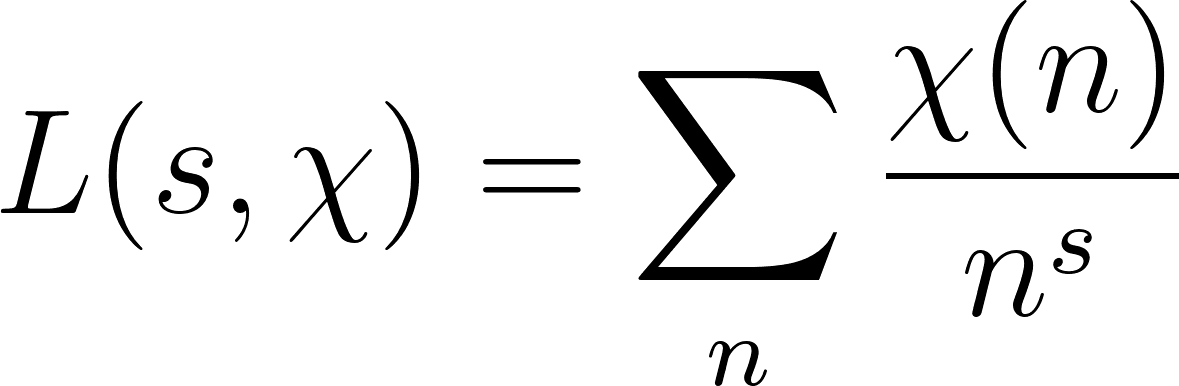Here, s is a complex number and Χ3 is a function that takes natural numbers as inputs and spits out complex numbers. (‘Σ’ is the summation symbol.)

The generalised Riemann hypothesis (GRH) is the conjecture that all zeros of the L-function L(s, Χ) that lie in the critical strip should also lie on the critical line. It’s the same hypothesis as before, reapplied to the L-function.

Now, a Landau–Siegel zero of the function L(s, Χ) is any real number between ½ and 1 that, when used for s, makes L(s, Χ) equal 0.

It is in effect a counterexample to the GRH: it implies that there could be a real number in the critical strip that doesn’t lie on the critical line, yet is a zero of the generalised zeta function.

Obviously, proving that there are no Landau-Siegel zeroes would be a weak form of proving the GRH. It wouldn’t allow us to claim that all non-trivial zeroes of the generalised function lie on the critical line – but it would allow us to say that some non-trivial zeroes definitely don’t lie outside the line.

Yitang Zhang’s preprint

On November 7, the Chinese-American mathematician Yitang Zhang announced that he had achieved a breakthrough in the study of Landau-Siegel zeroes. Specifically, he claimed to have proved a weaker version of the Landau-Siegel zeroes conjecture.

Note that this weakness is in addition to the weakness of the conjecture relative to the GRH – so the claim is in effect doubly weak. That said, it holds the hope of taking us closer to a highly complex and longstanding problem, so it merits our attention and scrutiny.

As number theorist Alex Kontorovich told Nature, “Resolving any of these issues would be a major advancement in our understanding of the distribution of prime numbers.”

Disproving the existence of Landau-Siegel zeroes requires mathematicians to prove that L(1, Χ) is much greater than (log D)-1.

In 2007, Zhang had published a preprint paper claiming that he had proved that L(1, Χ) was much greater than (log D)-17(log(log D))-1. But his proof turned out to be wrong after mathematicians noticed the incorrectness of a few key ideas developed in that paper.

In his new paper, Zhang claims to have proved that L(1, Χ) is much greater than (log D)-2022. He wrote about his work on a Chinese website called Zhihu, where he appears to claim that he tweaked his calculations to achieve the exponent to be 2022 – the year in which the result has been announced.

Zhang laboured in obscurity before he shot to fame in 2013 for his work on the twin-prime conjecture. He proved that there are infinitely many pairs of prime numbers separated by an even number that is lower than 70,000,000. The original conjecture is that there are infinitely many primes separated by an even number equal to 2. Zhang’s achievement was to bring this constant down from ‘finite but large’ to below 70 million.

The result was considered a great advancement. The Fields Medal winner Terence Tao initiated a large collaborative project called PolyMath8 to improve Zhang’s techniques and to lower the bound from 70 million. The 2022 Fields medallist James Maynard obtained a different proof of Zhang’s theorem which resulted in the project’s successful completion by lowering the bound to just 246.

It appears that Zhang did not use the ideas in his 2007 article in his new paper. Other mathematicians are only just beginning to review his work. Zhang has also said that his new techniques can be improved to lower the value of the exponent to the hundreds.

If Zhang’s claim is established, there is a chance that there will be another large PolyMath project to improve Zhang’s techniques. Even bringing the exponent down to the hundreds – as Zhang has said might be possible – won’t prove the Landau-Seigel zeroes conjecture but will be a significant advancement in the annals of number theory.

The reason is that there are several conjectures in fields as diverse as cryptography and quantum physics whose framing depends on the validity of the GRH. If GRH is proved, it will immediately also establish the correctness of all these other conjectures.

In many of these conjectures, the hypothesis can be replaced by a weak form of the Landau-Seigel conjecture of the type L(1,X) >> (log D)n, where n is any finite number. So if Zhang’s new result, with the -2022 exponent, is true, it will right away also prove these other conjectures.

Potential implications

One field where a resolution of the Riemann hypothesis will have a large effect is modern cryptography. A common encryption method involves an encryption key, which is public, and a decryption key, which is kept private. The decryption key is composed of two large prime numbers, and the encryption key is the product of these two numbers. Anyone can encrypt a message using the encryption key, but only the person holding the decryption key can decode it.

If the Riemann hypothesis is proved, it could lead to new techniques to find large prime numbers. This in turn could ease methods to factorise the encryption key into its two prime numbers, which would reveal the decryption key. Thus, cryptographers will have  to find a new way to secure information – one that doesn’t depend on prime-number factorisation – as system administrators scramble to secure sensitive data like user passwords, banking data, etc.

A solution to the Riemann hypothesis is also expected to open up beneficial applications. Quantum chaos is a subfield of quantum physics that studies quantum systems whose classical counterparts exhibit chaotic behaviour.

To borrow an example that Barry Cipra used in a 1998 article: It is easy to predict the path of a billiards ball rolling around in a rectangular space – and it’s equally easy when the ball is replaced with an electron. But when the ball is set in motion inside a pill-shaped box, its path becomes chaotic. Quantum chaos is concerned with predicting the path when the ball is replaced by an electron in the second case.

It uses a class of equations called trace equations – and it so happens that the Riemann zeta function has the form of a trace equation. This means a resolution to the Riemann hypothesis can help physicists create a quantum-chaotic system, like the electron in a pill-shaped box, and thus bring more order to the subfield and its widespread complexity.

A third potential implication (among several) is that the spacing of the zeroes of the Riemann zeta function roughly resembles the spacing between the energy levels of a heavy nucleus, such as erbium-166. Say you mark the energy of each of these levels as points on the number line. Then you derive the point-correlation function: it’s a function that tells you how many points on the line are separated by a distance d, where you get to pick d.

In 1972, the American mathematician Hugh Montgomery and the British physicist Freeman Dyson found that the pair-correlation function of the zeroes of the Riemann zeta function resembled the pair-correlation function used to describe the energy levels of a heavy nucleus.

This points to a deep connection between the Riemann zeta function and nuclear physics – and one that is emblematic of similar connections between the function and patterns in many branches of modern physics.

Mathematicians and physicists have interpreted these links to mean that the Riemann hypothesis ought to be true. But the only way Riemann’s conjecture can be proven once and for all is using the techniques of number theory. To this end, the new paper by Yitang Zhang offers another way forward.

With inputs from Vasudevan Mukunth.

Mohan R. is an assistant professor at Azim Premji University Bangalore. He works in the areas of mathematics communication and mathematics education.

1. 1 is neither prime nor composite

2. Pronounced ‘rho’

3. Pronounced ‘chi’

Scroll To Top